Function Repository Resource:

# BooleInverse

Inverse of the Boole function

Contributed by: Daniel Sanchez (Wolfram Research)
 ResourceFunction["BooleInverse"][expr] yields True if expr is 1 and False if it is 0.

## Details and Options

ResourceFunction["BooleInverse"][expr] remains unchanged if expr is neither 1 nor 0.
ResourceFunction["BooleInverse"][expr] is effectively equivalent to Switch[expr,1,True,0,False].
ResourceFunction["BooleInverse"] is Listable.

## Examples

### Basic Examples (2)

Apply BooleInverse to a 0 and a 1:

 In:=Out=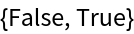Convert a list of 1s and 0s to truth values:

 In:=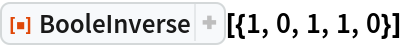Out=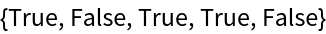### Applications (1)

BooleInverse is useful when testing a condition based on bit values:

 In:=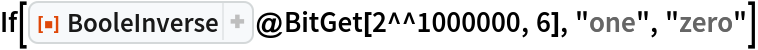Out=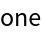### Properties and Relations (3)

BooleInverse[expr] is effectively equivalent to Switch[expr,1,True,0,False]:

 In:=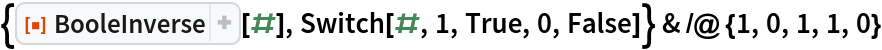Out=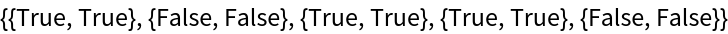BooleInverse is the inverse function of Boole:

 In:=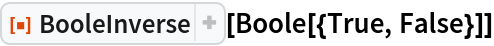Out=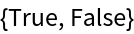BooleInverse deals with Rule in the same way that Boole does:

 In:=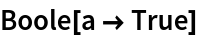Out=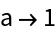In:=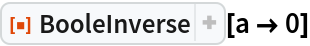Out=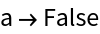### Possible Issues (2)

Only one argument is expected:

 In:=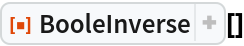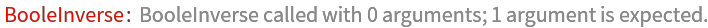Out=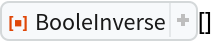In:=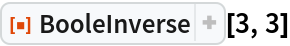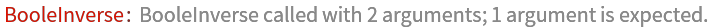Out=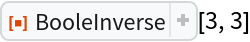If the sole argument provided is neither 0 nor 1, the expression is not evaluated:

 In:=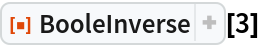Out=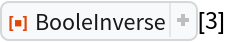Daniel Sanchez

## Version History

• 1.0.0 – 12 August 2020